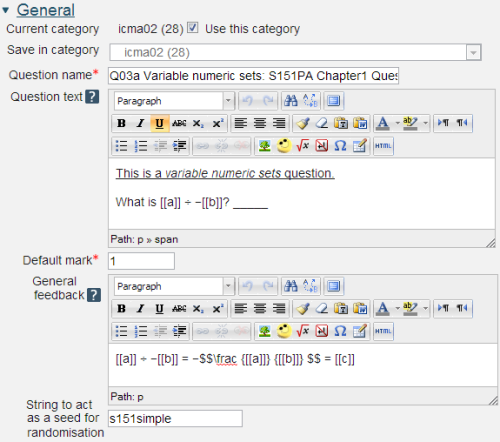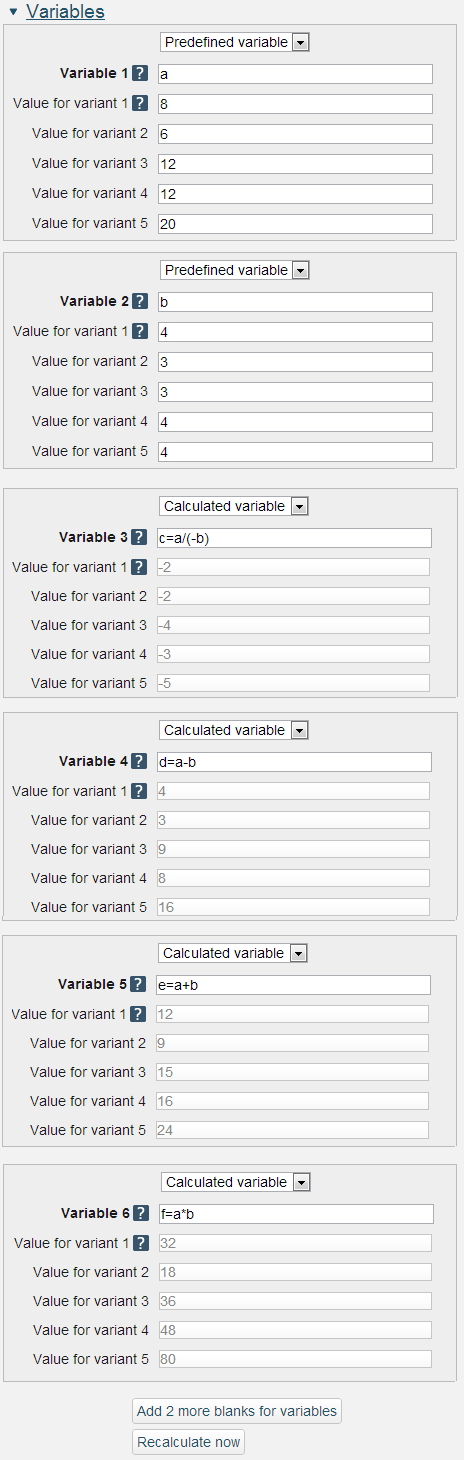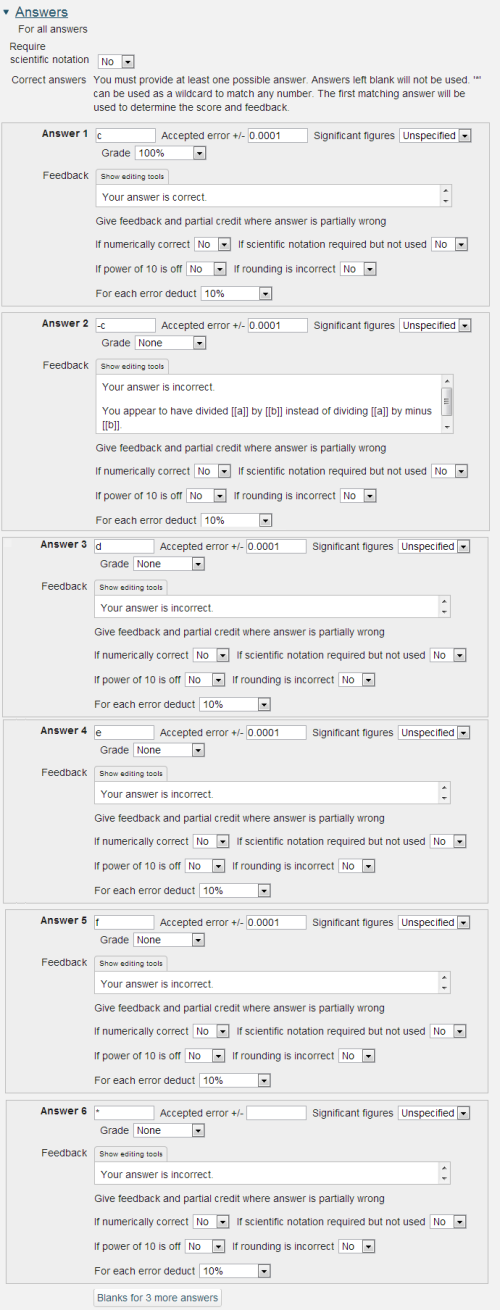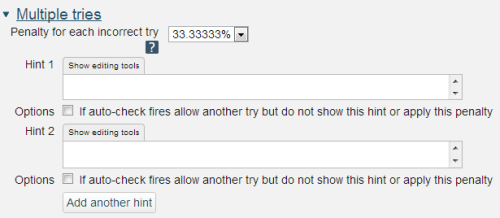# 2.1.3 Variable numeric sets

Variable numeric sets questions are similar to Variable numeric questions but are limited to ‘sets’ of pre-specified values. As such Variable numeric sets questions are safer as all possible variations can be tested before the question is released to students.

Variable numeric sets questions use numeric values and calculations that are performed at runtime following rules laid down in the question.2.12 The question, marks and general feedback for a variable numeric sets question

Question name: A descriptive name is sensible. This name will not be shown to students.

Question text: You may use the full functionality of the editor to state the question.

## Displaying the values of variables

Variables that are calculated by the computer are included in text fields by placing the names of the variables within double square brackets e.g. [[a]]. The formatting of the numbers may be controlled using sprinf() style controls (http://php.net/ manual/ en/ function.sprintf.php [Tip: hold Ctrl and click a link to open it in a new tab. (Hide tip)] ). For example [[a,.3e]] will display the value of a in scientific notation with 3 decimal places. The most usual formatting choices are ‘d’ for integers, ‘f’ for floating point or ‘e’ for scientific notation.

You may also include formatted mathematics using TeX or MathML. The text surrounded by two \$ symbols in this example will be interpreted by the TeX filter and converted to nicely formatted mathematics. Please see the Including TeX and MathML section below.

## Placing the response box

The response box may be placed within the question rubric by including a sequence of underscores e.g. _____. At runtime the response box will replace the underscores and the length will be determined by the number of underscores. The minimum number of underscores required to trigger this action is 5. If no underscores are present the response box will be placed after the question and will be a full length box.

General feedback: We recommend that all questions should have this box completed with the correct answer and a fully worked explanation. The contents of this box will be shown to all students irrespective of whether their response was correct or incorrect. We do not recommend that authors rely on using the machine generated 'Right answer' (from the iCMA definition form).

String to act as seed for randomisation: Sometimes it is desirable to base a sequence of questions on the same set of numbers. To do this use the same sets of numbers and the same string e.g. ‘mystring’ as the ‘seed for randomisation’ in each question in the sequence.

Please note that this then selects the same set of numbers across a sequence of questions for a student but different students get different sets of numbers.2.13 Two pre-specified sets of values and four calculated sets

## The names of variables must be lower case

As shown on this page variable names should use lower case letters.

The formula for all calculations should start with a variable name followed by an equals (=) sign and may use common mathematical operators +, -, *, /, brackets, (, ) and other variables defined within the question.

The mathematical processing that is available is similar to that provided within the Moodle Gradebook and includes

• average(a, b…): Returns the average of a list of arguments.
• max(a, b...): Returns the maximum value in a list of arguments
• min(a,b...): Returns the minimum value in a list of arguments
• mod(dividend, divisor): Calculates the remainder of a division.
• ln(number): Returns the natural logarithm of number.
• log(number): Returns the natural logarithm of number.
• pi(): Returns the value of the number Pi.
• power(base,exponent): Calculates the value of the first argument raised to the power of the second argument.
• round(number, count): Rounds a number to a predefined accuracy.
• sqrt, abs, exp
• sin, sinh, arcsin, asin, arcsinh, asinh
• cos, cosh, arcos, acos, arccosh, acosh
• tan, tanh, arctan, atan, arctanh, atanh

Recalculate now: With all variables specified the ‘recalculate now’ button will calculate a set of values within the greyed out boxes.2.14 The answers section

Require scientific notation: It is possible to require the student to enter their response using scientific notation. The input box is provided with a superscript facility and all of the following formats are accepted: 1.234e5, 1.234x105, 1.234X105 and 1.234*105.

For keyboard users the superscript may be accessed with the up-arrow key. Down-arrow returns entry to normal. You may wish to include this information within your question. If you do please note that the up-arrow and down-arrow provided in the HTML editor’s ‘insert custom characters’ list are not spoken by a screen reader and you should also include the words ‘up-arrow’ and ‘down-arrow’.

Answer: This may be a numeric value, a variable as above or a mathematical expression. For the example shown here it would have been quite acceptable to place ‘a/(-b)’ in the Answer box.

Accepted error: Computers store numbers to a finite accuracy e.g. 1/3 is stored as a finite number of recurring 3s in 0.33333… When used in calculations these minor infelicities can soon propagate and as such we strongly recommend that answers are matched within a suitable numerical range as shown here.

Significant figures: It is possible to request that responses be given to a specified number of significant figures.

## Dealing with responses that are partially wrong

Typically these are responses that have a variation on the correct numerical value e.g.

• are not in scientific format when this has been requested
• have an incorrect number of significant figures
• are wrong by a factor of 10
• have been rounded incorrectly.

In all of these four cases specific feedback can be supplied using these settings.2.15 Hints for use in Interactive with multiple tries behaviour

If you are configuring your question to run in 'Interactive with multiple tries' mode such that students can answer questions one by one you can also provide hints and second (and third) tries by entering appropriate feedback into the boxes provided in the 'Multiple tries' section of the question definition form.

To use this section of the question form please ensure that on the iCMA definition form you have chosen 'How questions behave' = 'Interactive with multiple tries'.

Penalty for each incorrect try: The available mark is reduced by the penalty for second and subsequent tries. In the example above a correct answer at the second try will score 0.6666667 of the available marks and a correct answer at the third try will score 0.3333334 of the available marks.

Hint: You can complete as many of these boxes as you wish. If you wish to give the student three tries at a question you will need to provide two hints. At runtime when the hints are exhausted the question will finish and the student will be given the general feedback and the question score will be calculated.

N.b. If you create a hint and then decide to delete it such that there is one less hint to the question you must ensure that all HTML formatting is also removed. To do this click on the 'HTML' icon in the editor toolbar which will display the HTML source. If any HTML formatting (in '< >') is present delete it and click on the HTML icon again to return to normal view.

2.1.2 Variable numeric

2.1.4 Variable numeric sets with units